• 讨论了常利率带干扰的多险种多复合Poisson-Geometric风险模型,推导出生存概率满足的积分-微分方程。在没有保费收入的情况下,得到生存概率的Laplace变换的表达式,并给出数值计算的实例以说明所得结果。
• 积分变换试海南大学信息、理工学院 《工程数学》试题(A卷) 课程代码： 考试时间：120分钟 得分 评卷人 1．某人打靶3发，事件Ai 表示“击中i发”，i=0,1,2,3. 那么事件A=A1∪A2∪A3表示( )。 A. 全部击中. B. ...
• 概率预测的目标是在满足 calibration 的前提下尽可能提高预测的 sharpness。所谓的 calibration 指的是预测分布和...1. 概率积分变换（Probability Integral Transform，PIT） 对于观测值 ξ1,⋯ ,ξn\xi_1, \cdot...

本文链接：个人站 | 简书 | CSDN
版权声明：除特别声明外，本博客文章均采用 BY-NC-SA 许可协议。转载请注明出处。

概率预测的目标是在满足 calibration 的前提下尽可能提高预测的 sharpness。所谓的 calibration 指的是预测分布和观测值在统计上的一致性，而 sharpness 则是指预测分布的集中程度。下面介绍一些常见的概率预测的评估方法。
1. 概率积分变换（Probability Integral Transform，PIT）
对于观测值 $\xi_1, \cdots, \xi_n$ ，假设模型预测的累积分布函数分别为 $F_1, \cdots, F_n$。如果模型预测准确，则概率积分变换 $\{F_i(\xi_i)\}_{i=1}^n$ 应当服从标准的均匀分布 $U(0,1)$。
PIT 的优势之一是便于可视化。最简单的做法是画直方图。$\cup$ 形的直方图意味着预测的分布过于集中；$\cap$ 形的直方图意味着预测的分布过于分散；明显不对称的直方图则意味着预测的分布整体偏离真实值。
import numpy as np
import matplotlib.pyplot as plt
import seaborn as sns
from scipy.stats import norm

sns.set()

obs = np.random.normal(loc=0, scale=1, size=1000)  # 观测值
pit_1 = norm.cdf(x=obs, loc=0, scale=1)  # 准确的预测
pit_2 = norm.cdf(x=obs, loc=0, scale=0.5)  # 预测过于集中
pit_3 = norm.cdf(x=obs, loc=0, scale=2)  # 预测过于分散
pit_4 = norm.cdf(x=obs, loc=1, scale=1)  # 均值偏离

plt.figure(figsize=(10, 8))

ax1 = plt.subplot(221)
sns.distplot(pit_1, bins=20, kde=False, color='g')
ax1.set_title('Histogram of PIT_1')

ax2 = plt.subplot(222)
sns.distplot(pit_2, bins=20, kde=False, color='g')
ax2.set_title('Histogram of PIT_2')

ax3 = plt.subplot(223)
sns.distplot(pit_3, bins=20, kde=False, color='g')
ax3.set_title('Histogram of PIT_3')

ax4 = plt.subplot(224)
sns.distplot(pit_4, bins=20, kde=False, color='g')
ax4.set_title('Histogram of PIT_4')

plt.tight_layout()
plt.show()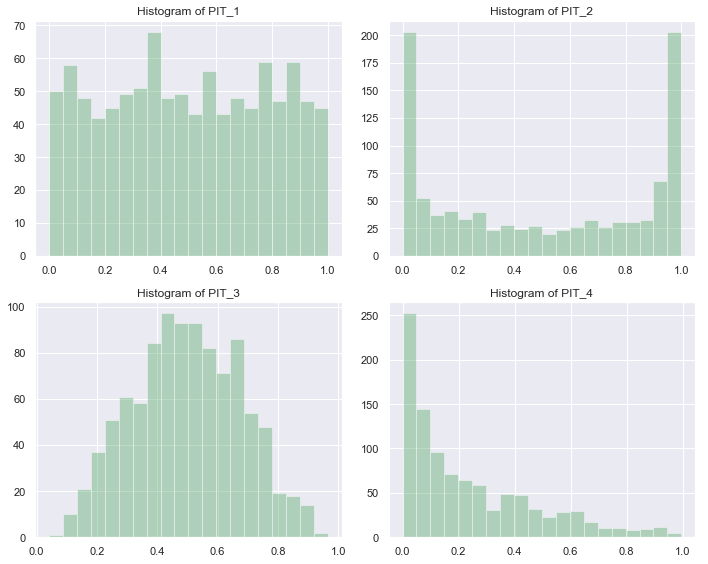PIT 还可以用 P-P 图来展示。简单地说，就是画出 PIT 的 CDF 与标准均匀分布的 CDF 的关系图。如果预测准确，得到的应该是一条直线。反 sigmoid 曲线意味着预测的分布过于集中；sigmoid 曲线意味着预测的分布过于分散；其它曲线则意味着预测的分布可能已经整体偏离真实值了。
from scipy.stats import uniform

def get_pp(pit, bins):
hist, edges = np.histogram(pit, bins, range=(0,1))
cdf = np.cumsum(hist) / np.sum(hist)
cdf_u = uniform.cdf(x=edges[1:])
return cdf_u, cdf

plt.figure(figsize=(10, 8))

ax1 = plt.subplot(221)
plt.plot(*get_pp(pit_1, 20), '-o')
ax1.set_title('PP plot of PIT_1')

ax2= plt.subplot(222)
plt.plot(*get_pp(pit_2, 20), '-o')
ax2.set_title('PP plot of PIT_2')

ax3 = plt.subplot(223)
plt.plot(*get_pp(pit_3, 20), '-o')
ax3.set_title('PP plot of PIT_3')

ax4 = plt.subplot(224)
plt.plot(*get_pp(pit_4, 20), '-o')
ax4.set_title('PP plot of PIT_4')

plt.tight_layout()
plt.show()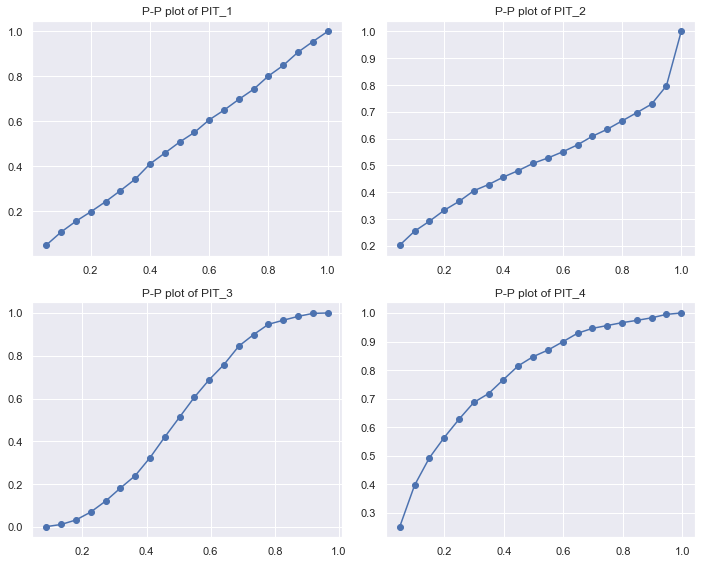2. 数值评分规则
2.1 连续概率排位分数（Continuous Ranked Probability Score，CRPS）
CRPS 是在概率预测领域使用最广泛的准确度指标之一。它的定义如下：
$CRPS(F^f, F^o) = \int_{-\infty}^{+\infty}\left[F^f(x)-F^o(x)\right]^2\mathrm dx \tag 1$
其中 $F^f$ 是预测分布的 CDF，$F^o$ 是观测值的 CDF。注意是平方的积分，千万不要误解为等于下图两条曲线之间的面积！！！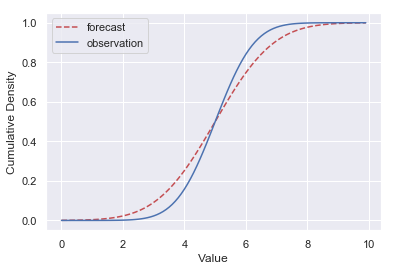由定义可知，CRPS 衡量的是预测分布和真实分布的差异，当预测分布与真实分布完全一致时，CRPS 为零。预测分布过于集中、过于分散，亦或是偏离观测值太远都会导致 CRPS 增大。
多数情况下，真实分布是未知的。如果对一系列的观测值 $\xi_1, \cdots, \xi_n$ 有各自对应的概率预测 $F_1, \cdots, F_n$，则可以用下式来估计 CRPS：
KaTeX parse error: No such environment: split at position 8:
\begin{̲s̲p̲l̲i̲t̲}̲
CRPS(F, \xi) &…
其中
$\varepsilon(t)= \begin{cases} 0, \qquad t<0\\ 1, \qquad t\geq0 \end{cases} \tag 3$
为单位阶跃函数，如下图所示。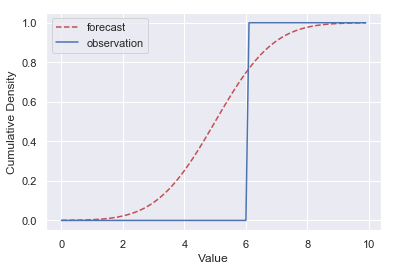2.2 交叉熵（Cross Entropy）和对数分数（Logarithmic Score）
如前所述，CRPS 衡量的是预测分布与真实分布之间的差异。我们知道，机器学习分类问题中常用的损失函数交叉熵也是用来比较两个概率分布之间的差异的。
概率分布 $p$ 和 $q$ 的交叉熵定义为
$H(p, q) = \mathbb E_p[-\log_2q] \tag 4$
其中 $p$ 为真实分布，$q$ 为预测分布。
若 $p$ 和 $q$ 是离散的，则
$H(p, q) = -\sum_xp(x)\log_2q(x) \tag 5$
在真实分布未知的情况下，可以用下式来估计交叉熵：
$H = -\frac1n\sum_{i=1}^n\log_2q(\xi_i) \tag 6$
其中 $\{\xi_i\}_{i=1}^n$ 为观测值。
如果对一系列的观测值 $\xi_1, \cdots, \xi_n$ 有各自对应的概率预测 $F_1, \cdots, F_n$，则对数分数（Logarithmic Score）定义为
$LogS(F, \xi) = -\frac1n\sum_{i=1}^n\log_2f_i(\xi_i) \tag 7$
其中 $f$ 为 $F$ 对应的 PDF。可以看到对数分数与交叉熵的估计式(6)形式上是相近的。
2.3 Brier Score
Brier Score 通常用于分类问题中，其定义为
$BS = \frac1n\sum_{t=1}^n\sum_{i=1}^r(f_{ti}-o_{ti})^2 \tag 8$
其中 n 是样本数量，r 是类目数量，$f_{ti}$ 是模型预测第 t 个样本的类目为 i 的概率，$o_{ti}$ 是第 t 个样本的真实状态（类目为 i 则取 1，否则取 0）。
3. 需要注意的问题
如前所述，真实分布已知的情况下，CRPS 可以直接计算。根据定义(1)，预测准确（即预测分布与真实分布完全一致）时 CRPS 为零。但真实分布未知的情况下，CRPS 只能通过(2)估算。此时就算预测准确，CRPS 也不为零。且不同的真实分布，在同样预测准确的时候，对应的 CRPS 也不一样。下面给出一个简单的例子：
>>> import numpy as np
>>> import properscoring as ps
>>> obs1 = np.random.normal(loc=0, scale=1, size=1000) # 从均值为0，方差为1的正态分布中采样作为观测值
>>> crps1 = np.mean(ps.crps_gaussian(x=obs1, mu=0, sig=1)) # 预测分布同样是均值为0，方差为1的正态分布，估算 CRPS 值
>>> crps1
0.5795829266550281
>>> obs2 = np.random.normal(loc=0, scale=10, size=1000) # 从均值为0，方差为10的正态分布中采样作为观测值
>>> crps2 = np.mean(ps.crps_gaussian(x=obs2, mu=0, sig=10)) # 预测分布同样是均值为0，方差为10的正态分布，估算 CRPS 值
>>> crps2
5.326040950564251
>>>

不能因为 crps1 比 crps2 小，就认为前者的预测更好，事实上它们都是对各自观测值真实分布的准确预测，因此是一样好的。在真实分布未知的情况下，CRPS 只适合用来衡量对同一个分布的不同预测之间的相对好坏，而不能衡量绝对的好坏。不难验证交叉熵也是如此。这与点估计中用到的各种准确率指标是不一样的。
怎样才能评估绝对的好坏呢？前面说过，预测准确的情况下，PIT 服从标准的均匀分布。如果计算 PIT 与标准均匀分布之间的 CRPS 或交叉熵，无论真实分布是怎样的，只要预测准确，结果都应该是接近的。
但 PIT 本身就没有问题了吗？如下图所示。左边两图中的红色实线表示真实值，绿色阴影表示预测的分布（采用均匀分布）。右边两图是对应的 PIT。从 PIT 得出的结论应该是上面的预测好，但上面这个真的是你需要的预测吗？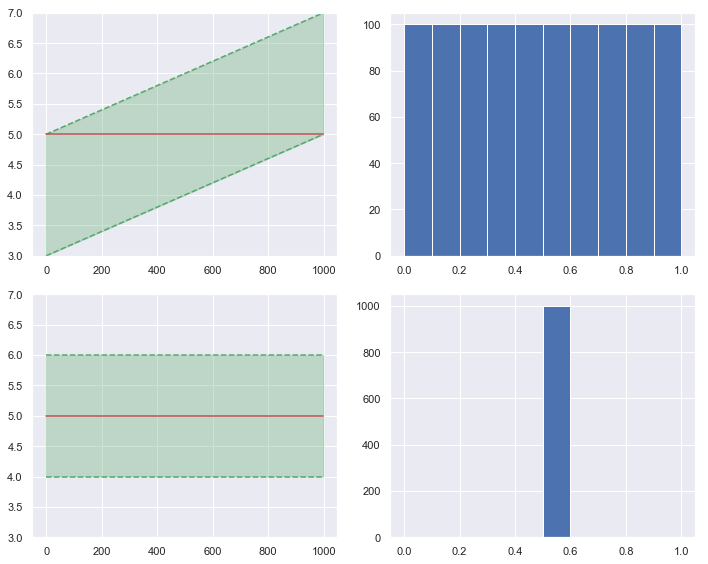参考文献
 Gneiting, T., & Raftery, A. E. (2007). Strictly proper scoring rules, prediction, and estimation. Journal of the American Statistical Association, 102(477), 359–378. https://doi.org/10.1198/016214506000001437
 Friederichs, P., & Thorarinsdottir, T. L. (2012). Forecast verification for extreme value distributions with an application to probabilistic peak wind prediction. Environmetrics, 23(7), 579–594. https://doi.org/10.1002/env.2176
 Benedetti, R. (2010). Scoring Rules for Forecast Verification. Monthly Weather Review, 138(1), 203–211. https://doi.org/10.1175/2009MWR2945.1
 Cross entropy - Wikipedia https://en.wikipedia.org/wiki/Cross_entropy
 Barier score - Wikipedia https://en.wikipedia.org/wiki/Brier_score


展开全文• 高斯分布： $f(x) = \frac{1}{\sqrt{2\pi }\sigma }exp(-\frac{(x-\mu)^{2}}{2\sigma^{2}})$ ...一个高斯分布只需线性变换即可化为标准高斯分布，所以只需推导标准高斯分布概率密度的积分。由...

高斯分布：
$f(x) = \frac{1}{\sqrt{2\pi }\sigma }exp(-\frac{(x-\mu)^{2}}{2\sigma^{2}})$
标准高斯分布：
$f(x) = \frac{1}{\sqrt{2\pi } }exp(-\frac{x^{2}}{2})$

一个高斯分布只需线性变换即可化为标准高斯分布，所以只需推导标准高斯分布概率密度的积分。由：
$\int_{-\infty }^{+\infty }\frac{1}{\sqrt{2\pi } }exp(-\frac{x^{2}}{2})dx = \int_{-\infty }^{+\infty }\frac{1}{\sqrt{2\pi } }exp(-\frac{y^{2}}{2})dy$
得:
$\int_{-\infty }^{+\infty }\frac{1}{\sqrt{2\pi } }exp(-\frac{x^{2}}{2})dx\int_{-\infty }^{+\infty }\frac{1}{\sqrt{2\pi } }exp(-\frac{y^{2}}{2})dy = \frac{1}{2\pi}\int_{-\infty }^{+\infty }\int_{-\infty }^{+\infty }exp(-\frac{(x^{2}+y^{2})}{2})dxdy$
变换为极坐标得：
$x = rcos\theta , y = rsin\theta$
$\frac{1}{2\pi}\int_{-\infty }^{+\infty }\int_{-\infty }^{+\infty }exp(-\frac{(x^{2}+y^{2})}{2})dxdy = \frac{1}{2\pi}\int_{0}^{2\pi }\int_{0}^{+\infty }exp(-\frac{r^{2}}{2})rdrd\theta$
$\frac{1}{2\pi}\int_{-\infty }^{+\infty }\int_{-\infty }^{+\infty }exp(-\frac{(x^{2}+y^{2})}{2})dxdy = \int_{0}^{+\infty }exp(-\frac{r^{2}}{2})rdr$
令$t = \frac{r^{2}}{2}$,得：
$\int_{0}^{+\infty }exp(-\frac{r^{2}}{2})rdr = \int_{0}^{+\infty }exp(-t)dt = 1$
终得：
$\int_{-\infty }^{+\infty }\frac{1}{\sqrt{2\pi } }exp(-\frac{x^{2}}{2})dx = \int_{-\infty }^{+\infty }\frac{1}{\sqrt{2\pi } }exp(-\frac{y^{2}}{2})dy = 1$
由于标准高斯分布概率密度函数$f(x)$满足：
$\int_{-\infty }^{+\infty }f(x)dx = \int_{-\infty }^{+\infty }f(x-c)dx$
$\int_{-\infty }^{+\infty }f(x)dx = \frac{1}{a}\int_{-\infty }^{+\infty }f(\frac{x}{a})dx$
所以任意高斯分布概率密度函数积分为1。

高斯分布的均值：
$E(x) = \int_{-\infty }^{+\infty }\frac{1}{\sqrt{2\pi }\sigma }exp(-\frac{(x-\mu)^{2}}{2\sigma^{2}})xdx$
$= \int_{-\infty }^{+\infty }\frac{1}{\sqrt{2\pi }\sigma }exp(-\frac{(x-\mu)^{2}}{2\sigma^{2}})（x-\mu）dx + \mu\int_{-\infty }^{+\infty }\frac{1}{\sqrt{2\pi }\sigma }exp(-\frac{(x-\mu)^{2}}{2\sigma^{2}})dx$
由奇偶性得：
$E(x) = \mu\int_{-\infty }^{+\infty }\frac{1}{\sqrt{2\pi }\sigma }exp(-\frac{(x-\mu)^{2}}{2\sigma^{2}})dx = \mu$
高斯分布的方差：
$Var(x) = \int_{-\infty }^{+\infty }\frac{1}{\sqrt{2\pi }\sigma }exp(-\frac{(x-\mu)^{2}}{2\sigma^{2}})(x-\mu)^{2}dx$
$\int_{-\infty }^{+\infty }\frac{1}{\sqrt{2\pi }\sigma }exp(-\frac{y^{2}}{2\sigma^{2}})y^{2}dy = \sigma^{2}$

转载于:https://www.cnblogs.com/shenchuguimo/p/9843034.html
展开全文• 此函数使用概率积分变换生成根据拉普拉斯分布分布的随机数matlab
• 积分变换定理 设 fff 是 (Ω,F,μ)\left(\Omega, \mathscr{F}, \mu\right)(Ω,F,μ) 到 (E,E)\left(E, \mathscr{E}\right)(E,E) 的可测映射，定义 μf:=μ(f−1(B))\mu_f:=\mu(f^{-1}(B))μf​:=μ(f−1(B)), 由定理...
实数的覆盖
$\mathbb{R}^n$ 中任意开集可表成可列个互不相交的半开闭方体的并。把半开闭方体稍微加大一点，那么 $\mathbb{R}^n$ 中任意开集可表成可列个开矩体的并。
$\mathbb{R}^n$ 任意子集，可以找到可数个开集进行覆盖。
$\bigcup_{r_i \in \mathbb{Q}^n\cap E}B(r_i, \epsilon_0)$
结合以上两条，可以定义 $\mathbb{R}^n$ 任意子集的外测度，即所有可数开矩体的覆盖中取inf。
随机过程的定义
首先给出随机过程的一个简单的定义，
定义1：$\left(\Omega, \mathscr{F}, \mu\right)$，取一族可测空间 $(S_t, \mathscr{B}_t)$，一个随机过程可以看出一族随机变量 $X_t\ (t\in T)$，固定每个 $t_0$，$X_{t_0}:\Omega \rightarrow S_{t_0}$ 是可测函数。
用上面的定义，显然随机过程是存在的。但是由于人类不可能写出所有的这样的映射，如何构造一个随机过程形成了困难。我们想把 $X(t,\omega)$ 看成是一个随机变量 $X(\cdot, \omega)\in \underset{t \in T}{\prod} S_{t}$，记 $S_T:=\underset{t \in T}{\prod} S_{t}$
【这样想的原因是，由于随机变量把概率空间转化到可测空间 $(S_{t}, \mathscr{B}_t)\ t\in T$ 上，那么研究清楚 $(S_{t}, \mathscr{B}_t)\ t\in T$ 就可以知道所有随机变量能够表达的信息了。这个概率空间研究清楚后，概率空间的存在性就解决了，取 $(S_T, \mathscr{B}_T,\mu_T)$ 即可，按照原始的随机过程定义，取自然的投影映射，随机过程便存在了】
（Kolmogorov相容性定理） $(S_{t}, \mathscr{B}_t)\ t\in T$ 是一族Borel空间族，任意有限个的乘积空间的概率测度满足相容性，那么存在 $\left(\Omega, \mathscr{F}, \mu\right)$ 和随机过程  $X(t,\omega)$。
【采用定义1，如果要找出随机过程，虽然概率空间好找，但是需要手写无限个映射，这是不可能的。Kolmogorov相容性定理告诉我们，可以从另一个角度写出随机过程，只要写出有限维分布，满足相容性定理就可以了。这样，概率空间采用 $(S_T, \mathscr{B}_T,\mu_T)$，映射就是投影到$(S_{t}, \mathscr{B}_t,\mu_t)\ t\in T$，采用这种方法的代价是，概率空间就非常难写，并且一定要给出 $\mu_T$，因此Kolmogorov相容性定理的主要难点就是在找概率空间上。显然，概率空间找的就是 $(S_T, \mathscr{B}_T)$，但是他的测度就带来困难了。】
乘积空间
先采用定义1，那么概率空间和无限个映射均存在，挖掘一些性质。以下先讨论乘积空间如何建立可测空间和可测映射，先不讨论测度。
先建立无穷维乘积空间，开始等价定义转换，假设定义中的 $\sigma-$代数为 $\mathscr{B}_T$，要使得两个定义等价，需要
$\forall t \in T, X(t, \cdot)\in \mathscr{F}|\mathscr{B}_t \Leftrightarrow X(\cdot, \cdot)\in \mathscr{F}|\mathscr{B}_T$
取 $\mathscr{M}=\{B_{t_{0}}\times \underset{t \in T\backslash\{t_{0}\}}{\prod}S_t: t_0\in T, B_{t_{0}}\in \mathscr{B}_{t_{0}} \}$，$\mathscr{B}_T$ 的构造方法是 $\sigma(\mathscr{M})$。
$\Rightarrow$：要证 $X(\cdot, \cdot)\in \mathscr{F}|\mathscr{B}_T$，只需要证 $\forall B\in \mathscr{M},X^{-1}(B)\in \mathscr{F}$。这是显然的。
$\Leftarrow$：要证 $\forall t_0,X(t_0, \cdot)\in \mathscr{F}|\mathscr{B}_{t_{0}}$，看投影映射 $\pi_{T,t_{0}}y=y(t_0),y\in S_T$。$X(t_0,\omega)=\pi_{T,t_{0}}X(\omega)$。

下面证明，任意 $B\in \mathscr{B}_T$，存在可数子集 $T_c$，使得 $B=B_{T_c}\times \underset{t \in T\backslash\{t_{0}\}}{\prod}S_t$，其中 $B_{T_c}\in \mathscr{B}_{T_c}$，是可数的乘积空间。
由上述知，显然 $C[0,1]\notin \mathscr{B}_T$
【产生这种性质的原因是定义只要求在某一点满足可测性，再有 $\sigma$ 产生的方式导致只能做到可数个的情况】

乘积空间的测度
【2】有限维乘积空间的测度如何构造
如果有幸构造出转移概率，那么可以构造乘积空间的测度，例如马尔可夫过程，随机游走等…
如果已知乘积空间和各个空间的概率测度，那么也可以导出转移概率
【3】可列维乘积空间的测度
【4】无穷维乘积空间的测度
Tulcea定理的条件是任意有限 $n$，存在转移概率。
Kolmogorov相容性定理给出的条件是，乘积空间存在概率测度，因此可以找出转移概率，然后用Tulcea定理，存在可列维乘积空间概率测度，接着推广到整个空间上去。
由于Kolmogorov相容性的条件并不好找，转移概率也并不好找，因此随机过程说来说去也就那几种
积分定义
$f$ 为 $\left(\Omega, \mathscr{F}, \mu\right)$ 上非负可测函数，则 $f$ 积分的定义是
$\int_{\Omega} f(\omega) \mu(d\omega)=sup\left\{\int_{\Omega}h(\omega)\mu(d\omega):0\le h\le f, h \ simple \right\}.$
从定义上似乎不要求 $f$ 为可测函数，但是由于任何非负可测函数都存在递增的简单函数列进行逼近。如果 $f$ 不为可测函数，想象一下在某个不可测集上 $f$ 长得奇奇怪怪，任何简单函数都没办法逼近那个奇怪的东西，因此就干脆要求，能定义积分的函数都是可测函数了。
积分变换定理
设 $f$ 是 $\left(\Omega, \mathscr{F}, \mu\right)$ 到 $\left(E, \mathscr{E}\right)$ 的可测映射，定义 $\mu_f:=\mu(f^{-1}(B))$, 由定理【待补充】的证明知，$\mu_f$ 是 $\mathscr{E}$ 上的测度，称为 $\mu$ 在 $\mathscr{E}$ 上由 $f$ 导出的测度。
（积分变换定理）设 $f$ 是 $\left(\Omega, \mathscr{F}, \mu\right)$ 到 $\left(E, \mathscr{E}\right)$ 的可测映射，$g$ 是 $\left(E, \mathscr{E}\right)$ 上的可测函数（$\left(E, \mathscr{E}\right)$ 到 $\left(\mathbb{R}, \mathscr{B_{\mathbb{R}}}\right)$ ），则有：
$\int_{f^{-1}(B)} (g\circ f)(\omega) \mu(d\omega)=\int_{B} g(x) \mu_f(dx), \forall B \in \mathscr{E}.$
证明：【待补充】，思路是先看简单函数，慢慢推广。
分布函数
一个简单情况是，$X$ 是 $\left(\Omega, \mathscr{F}, \mu\right)$ 到 $\left(\mathbb{R}, \mathscr{B_{\mathbb{R}}}\right)$ 的随机变量，【待补充】分布函数 $F_X$ 是  $\mu$ 在 $\mathscr{B_{\mathbb{R}}}$ 上由 $X$ 导出的测度。由积分变换定理，
$\mu(X\in B)=\int_{X^{-1}(B)} \mu(d\omega)=\int_{B} F_X(dx), \forall B \in \mathscr{E}.$
$I$ 为恒等映射，则
$EX=\int_{\Omega} I\circ X(\omega)\mu(d\omega)=\int_{\mathbb{R}} xF_X(dx), \forall B \in \mathscr{E}.$
$g$ 为可测函数，则
$E[g(X)]=\int_{\Omega} g\circ X(\omega)\mu(d\omega)=\int_{\mathbb{R}} g(x)F_X(dx), \forall B \in \mathscr{E}.$
【从积分变换定理可以看出，随机变量的意义是把概率空间转化到实数上进行讨论。对于概率空间可测集的积分并不能直接算得。引入随机变量，而且这个随机变量通常是Borel可测的。概率空间上的测度导出对Borel可测集的测度，也就是分布，那么对随机变量的积分最后都化成了对实数上Borel可测集的积分】


展开全文• title: 【概率论】3-8:随机变量函数(Functions of a Random Variable) categories: Mathematic Probability keywords: The Probability Integral Transformation 概率积分变换 Simulation 仿真 Pseudo-Random...


title: 【概率论】3-8:随机变量函数(Functions of a Random Variable)
categories:
Mathematic
Probability
keywords:
The Probability Integral Transformation
概率积分变换
Simulation
仿真
Pseudo-Random Numbers
伪随机数
General Function
toc: true
date: 2018-03-16 09:49:24Abstract: 本文介绍通过函数这个工具，来研究随机变量Keywords: The Probability Integral Transformation，Simulation，Pseudo-Random Numbers，General Function

开篇废话
我们到目前为止对概率的研究经过了试验结果，事件，随机变量大概这三个过程，其实每个过程都是更高层的抽象，比如，对于直观的事实，实验结果，我们通过一种函数，或者称为一种收集，将结果抽象成了事件，而对事件研究了一段时间后又将事件通过函数（随机变量）映射到了实数域，整个过程，更加抽象，更加复杂，但是计算和模拟现实中的试验结果变得更加容易更加准确。
对于实数的研究，函数是绕不开的话题，而函数的微分积分等又是现代科学的基础，所以本文简要的介绍下随机变量的函数。
问题的描述变成了当我们已知一个随机变量 XX 具有某个p.f. 或者 p.d.f 那么随机变量 Y=f(x)Y=f(x) 分布是什么。
Random Variable with a Discrete Distribution
先看一个例子：
离散随机变量 XX 在 [1,…,9][1,\dots ,9] 有一个均匀分布，我们关系的是随机变量距离区间中心5的距离Y的分布情况。
这时候 YY 的定义的数学化表示是： Y=∣X−5∣Y=|X-5| 其分布函数不太好写，但是可以列举出来：Y∈{0,1,2,3,4}Pr(Y=1)=Pr(X∈4,6)=29Pr(Y=2)=Pr(X∈3,7)=29Pr(Y=3)=Pr(X∈2,8)=29Pr(Y=4)=Pr(X∈1,9)=29Pr(Y=0)=Pr(X∈5)=19
Y\in \{0,1,2,3,4\}\\
Pr(Y=1)=Pr(X\in {4,6})=\frac{2}{9}\\
Pr(Y=2)=Pr(X\in {3,7})=\frac{2}{9}\\
Pr(Y=3)=Pr(X\in {2,8})=\frac{2}{9}\\
Pr(Y=4)=Pr(X\in {1,9})=\frac{2}{9}\\
Pr(Y=0)=Pr(X\in {5})=\frac{1}{9}\\

这就是一个最简单的例子，关于离散随机变量的函数的分布问题。

Theorem Function of a Discrete Random Variable. Let XX have a discrete distribution with p.f. ff and let Y=r(X)Y=r(X) for some function of rr defined on the set of possible values of XX For each possible value y of YY the p.f. gg of YY isg(y)=Pr(Y=y)=Pr[r(X)=y]=∑x;r(x)=yf(x)
g(y)=Pr(Y=y)=Pr[r(X)=y]=\sum_{x;r(x)=y}f(x)

解读下上面的公式，其实公式写的很清楚，当我们知道函数r了以后，满足 r(X)=yr(X)=y 的所有X对应的概率最后组合成了Y，所以要进行求和，其实这一步跟从试验结果得到事件的过程是一样的。但是下面对于连续分布来说，就非常不一样了。
以上为节选内容，完整原文地址：https://www.face2ai.com/Math-Probability-3-8-Fuctions-of-a-Random-Variable转载请标明出处

转载于:https://www.cnblogs.com/face2ai/p/9756543.html
展开全文• ## 抽样方法

千次阅读 2015-05-03 14:34:49
来自于中科院《统计学习基础》研究生课程第9-13讲 概率积分变换方法 basic monte carlo method acceptance-rejection method 重要性采样 MCMC统计学 MCMC
• 由于保险公司经营规模的扩大和保险业务经营受货币利率的...我们构造了常利率下两险种的风险模型，利用后项微分法和Laplace变换，给出了破产概率Ψδ(u)的积分方程和破产概率Laplace变换表达式，以及Ψδ(0)的确切值.
• 任意分布随机数生成概率积分变换取舍法 概率积分变换 若X为连续型随机变量，其cdf为FX，则 U=FX−1(X)∼U(0,1) U=F^{-1}_X(X) \sim U(0,1) U=FX−1​(X)∼U(0,1) 证明： FX(X=u)=P(X≤u)FX−1(u)={x∣FX(x)=u}=...统计学
• 引入双险种,且保险收取与索赔过程服从二项分布,利用等价变换及全概率公式得到模型的破产概率积分表达式,通过鞅方法证明改进后的模型存在破产概率上界,并应用停时定理证明该破产概率上界优于不带投资情况下的破产概率...
• 本文考虑一类具有两个独立险种的风险模型的...在索赔计数过程是普通Erlang(2)过程的情形下，证明了广义Lundber9方程有且仅有三个正的实数根，由此并结合破产概率满足的积分-微分方程组，给出了破产概率的Laplace变换
• 我们描述了一个质量分数，它可以根据概率积分变换的统计一致性对概率密度预测进行排序（Diebold 等人，1998b）。 然后，我们描述了一个框架，可以根据任何目标函数集优化任何密度预测模型。 该框架使用多目标进化...
• 雅可比变换公式的推到 就是要求这个面积系数 类似于这样的变量积分都可以用变量代换算 这个是先证明局部，再证明整体 转载于:https://www.cnblogs.com/china520/p/10751577.html...
• 基于AD+FPGA的硬件架构完成了带宽为200 MHz的跳频和直接序列扩频混合扩频信号的解跳、解扩，实现了基于Xilinx的快速傅里叶变换IP核的扩频码的快速滑动码相关，以及多跳积分结果的相干累加，并采用相对门限法进行了...FPGA
• 高斯积分是在概率论和连续傅里叶变换等的统一化等计算中有广泛的应用。...高斯积分（Gaussian integral），有时也被称为概率积分，是高斯函数的积分。它是依德国数学家兼物理学家卡尔·弗里德里希·高斯之姓氏所命名。
• 最直接的方法是基于概率积分变换属性：如果 $U$ 在 $(0,1)$ 上均匀分布，则 $Φ−1(U)$ 将具有标准正态分布。 这种方法的缺点是它依赖于概率函数 $Φ−1$ 的计算，而这不能通过解析来完成。 一种依赖于中心极限...C++
• 与CDT相似，Radon-CDT也源于最佳质量传输的丰富数学基​​础，并将其与积分几何和Radon变换相结合。 与当前数据广泛的非线性模型（包括深层神经网络及其变体）不同，Radon-CDT提供了定义明确的可逆非线性变换，可与...
• 3连续型随机变量的生成: 3.1反函数法 采用概率积分变换原理,对于随机变量X的分布函数F(X)可以求其反函数，得:Xi=G(Ri)其中,Ri为一个0-1区间内的均匀分布的随机变量.F(X)较简单时，求解较易，当F(X)较复杂时，需要...matlab 算法 float output
• 在这封信中，提出了一种新颖的长期相干积分方法，称为Radon线性规范变换（RLCT），用于检测海杂波中... 最后，利用真实海杂波数据集进行的实验表明，该方法能够在重海杂波中获得较高的积分增益和微运动目标的检测概率
• 传统的积分是在区间上进行的，后来人们希望把积分推广到任意的集合上，就发展出测度的概念，它在数学分析和概率论有重要的地位。 测度的具体定义为(还是来自百度百科) 定义1：构造一个集函数，它能赋予实数集簇......